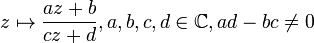# Projective special linear group:PSL(2,C)

View a complete list of particular groups (this is a very huge list!)[SHOW MORE]

## Definition

This group, denoted$PSL(2,\mathbb{C})$ or$PGL(2,\mathbb{C})$, is defined in the following equivalent ways:

1. It is the projective special linear group of degree two over the field of complex numbers.
2. It is the projective general linear group of degree two over the field of complex numbers.

### As a group of conformal automorphisms

The group$PSL(2,\mathbb{C})$ is the automorphism group of the Riemann sphere. Explicitly, viewing the Riemann sphere as$\mathbb{C} \cup \{ \infty \}$, its automorphisms are given as fractional linear transformations of the form:$z \mapsto \frac{az + b}{cz + d}, a,b,c,d \in \mathbb{C}, ad - bc \ne 0$

The composition of these works like multiplication of the corresponding matrices$\begin{pmatrix} a & b \\ c & d \\\end{pmatrix}$.

The reason why the group is the projective special linear group rather than the general linear group is that matrices that are scalar multiples of each other define the same fractional linear transformation, so we need to quotient out by the center. The fact that$PSL(2,\mathbb{C})$ is the same as$PGL(2,\mathbb{C})$ follows from the fact that every nonzero element of$\mathbb{C}$ is the square of a nonzero element (this follows from$\mathbb{C}$ being algebraically closed).

From an algebraic perspective, if we think of the Riemann sphere as$\mathbb{P}^1(\mathbb{C})$, the action by fractional linear transformations is just the usual way any projective special linear group of degree two over a field acts on the corresponding projective line.

Note: It requires a bit of complex analysis to show that the only conformal automorphisms possible for the Riemann sphere are the ones described by fractional linear transformations.

### Structures

The group can be viewed at many levels of structure:

## Arithmetic functions

Function Value Similar groups Explanation
dimension of an algebraic group 3 As$PSL(n,\_), n = 2$:$n^2-1 = 2^2-1 = 3$ (note that this dimension is considering the group as an algebraic group over$\mathbb{C}$. The dimension as an algebraic group over$\R$ is twice this dimension, i.e., 6.
dimension of a complex Lie group 3 As$PSL(n,\mathbb{C}), n = 2$:$n^2-1 = 2^2-1 = 3$
dimension of a real Lie group 6 Twice the dimension as a complex Lie group.

## Group properties

Property Satisfied? Explanation
simple group, simple non-abelian group Yes projective special linear group is simple (with exceptions$PSL(2,2)$ and$PSL(2,3)$)
abelian group No
nilpotent group No
solvable group No

## Elements

Further information: element structure of projective special linear group:PSL(2,C)

## Subgroups

Further information: subgroup structure of projective special linear group:PSL(2,C)

Closed subgroups that are discrete under the subspace topology are termed Kleinian groups.